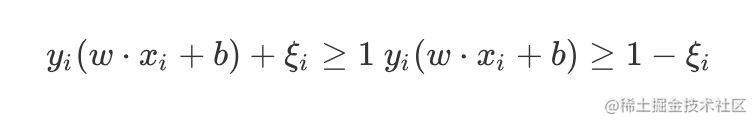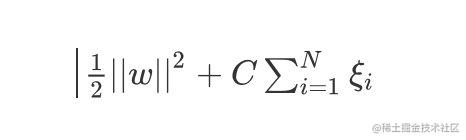# 吴恩达机器学习-7-支持向量机SVM

• 硬间隔
• 支持向量
• 软间隔
• 对偶问题

### 优化目标Optimization Objectives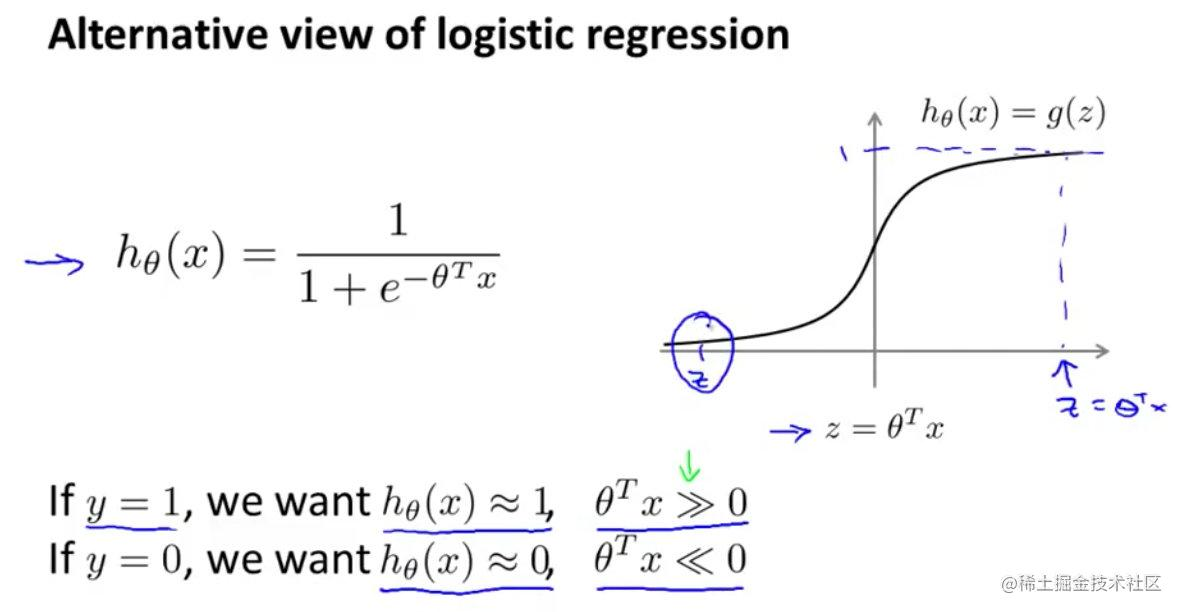• 左边是假设函数
• 右边是Sigmoid激活函数

$z={\theta}^{T}x$，如果满足：

1. $y=1$，希望$h{(\theta)}$约为1，将样本正确分类，那么z必须满足$z>>0$
2. $y=0$，希望$h(\theta)$约为0，将样本正确分类，那么z必须满足$z<<0$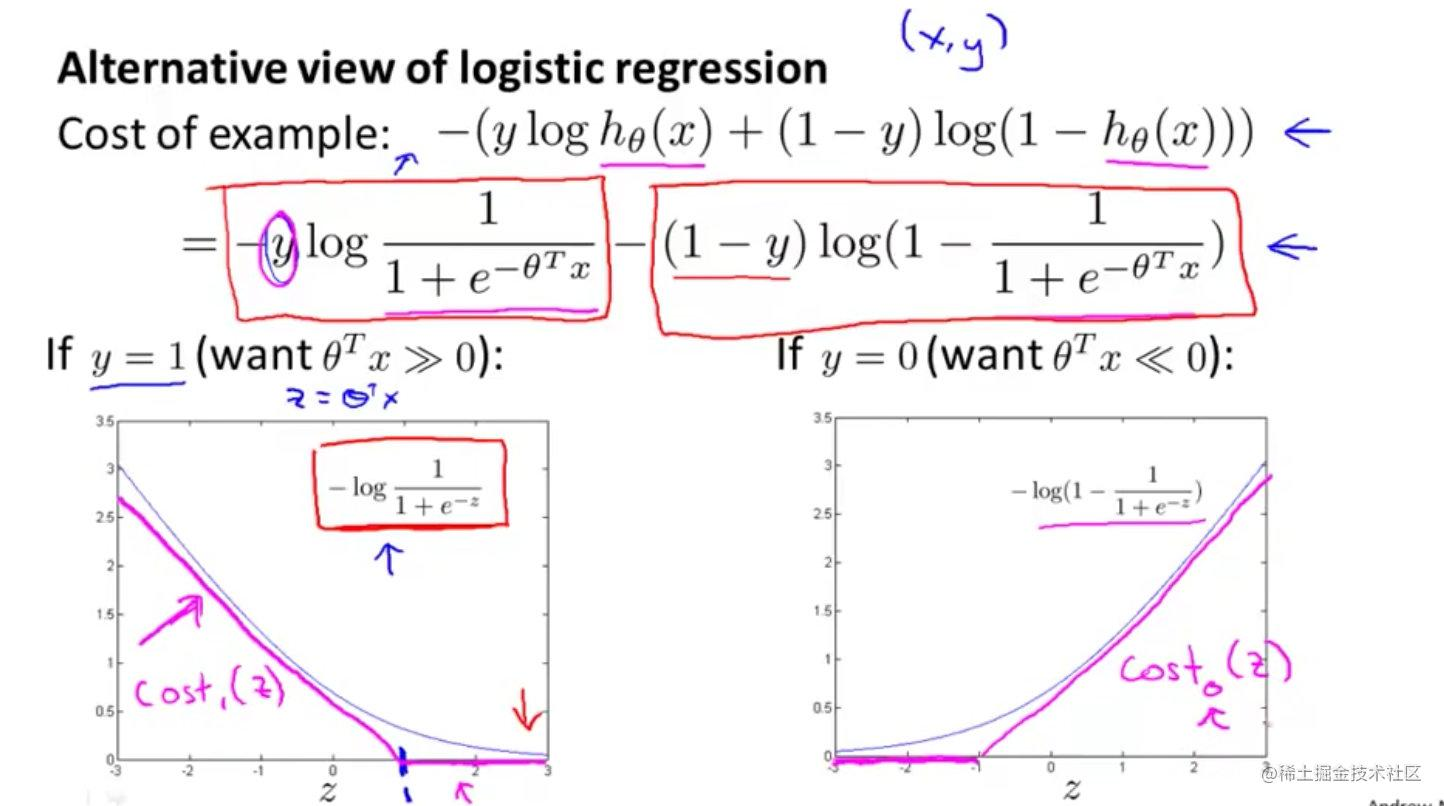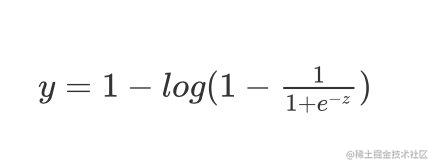$y=1-log(1-\frac{1}{1+e^{-z}})$

### 支持向量机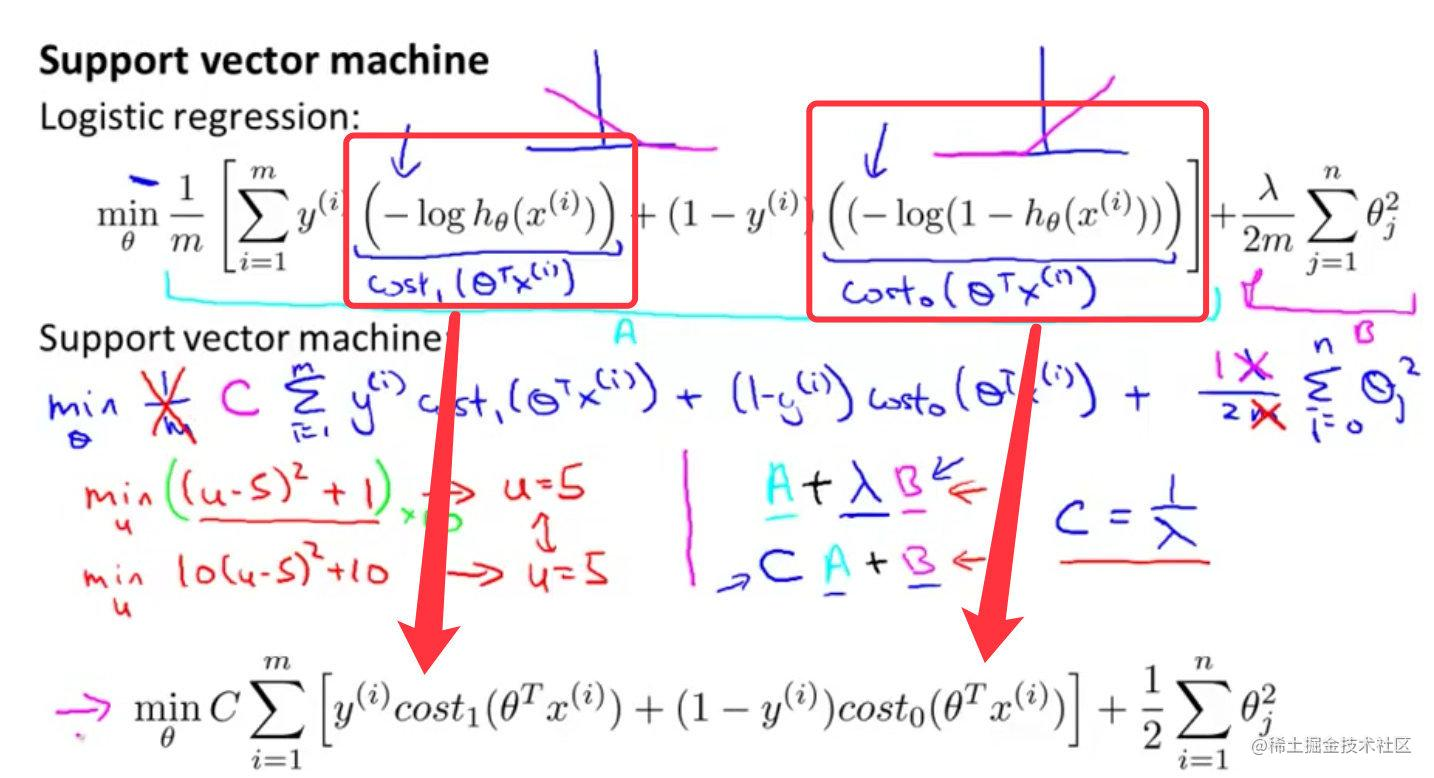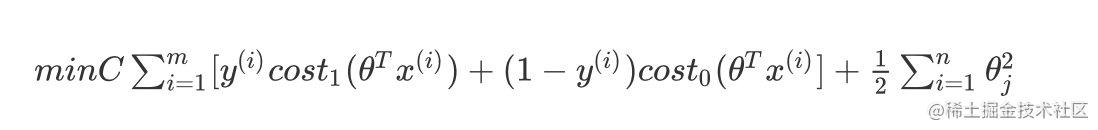$min C\sum^m_{i=1}[y^{(i)}cost_1(\theta^Tx^{(i)})+(1-y^{(i)})cost_0(\theta^Tx^{(i)}]+\frac{1}{2}\sum^n_{i=1}\theta_{j}^2$

• 第一个是训练样本的代价
• 第二个是正则化项

### 大边界的直观解释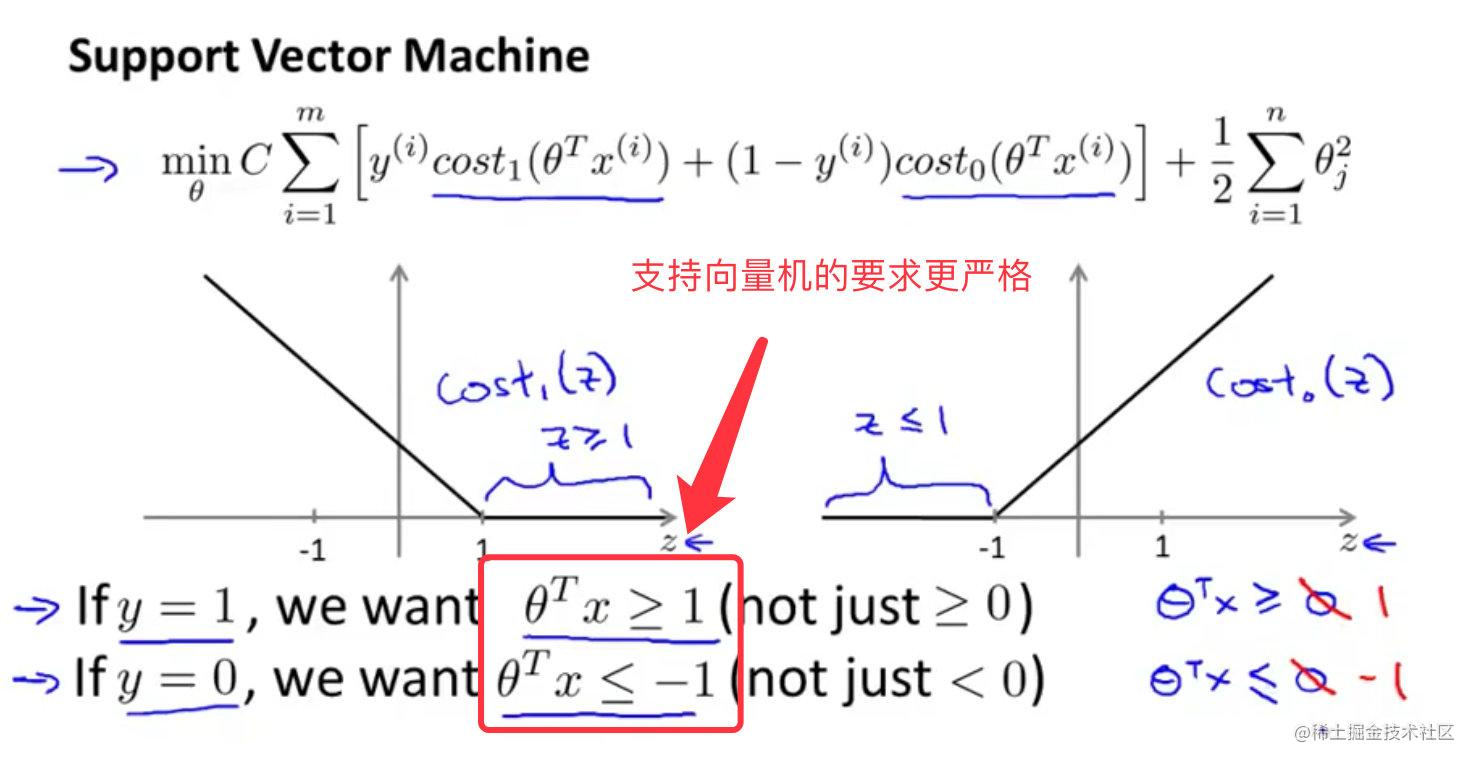#### SVM决策边界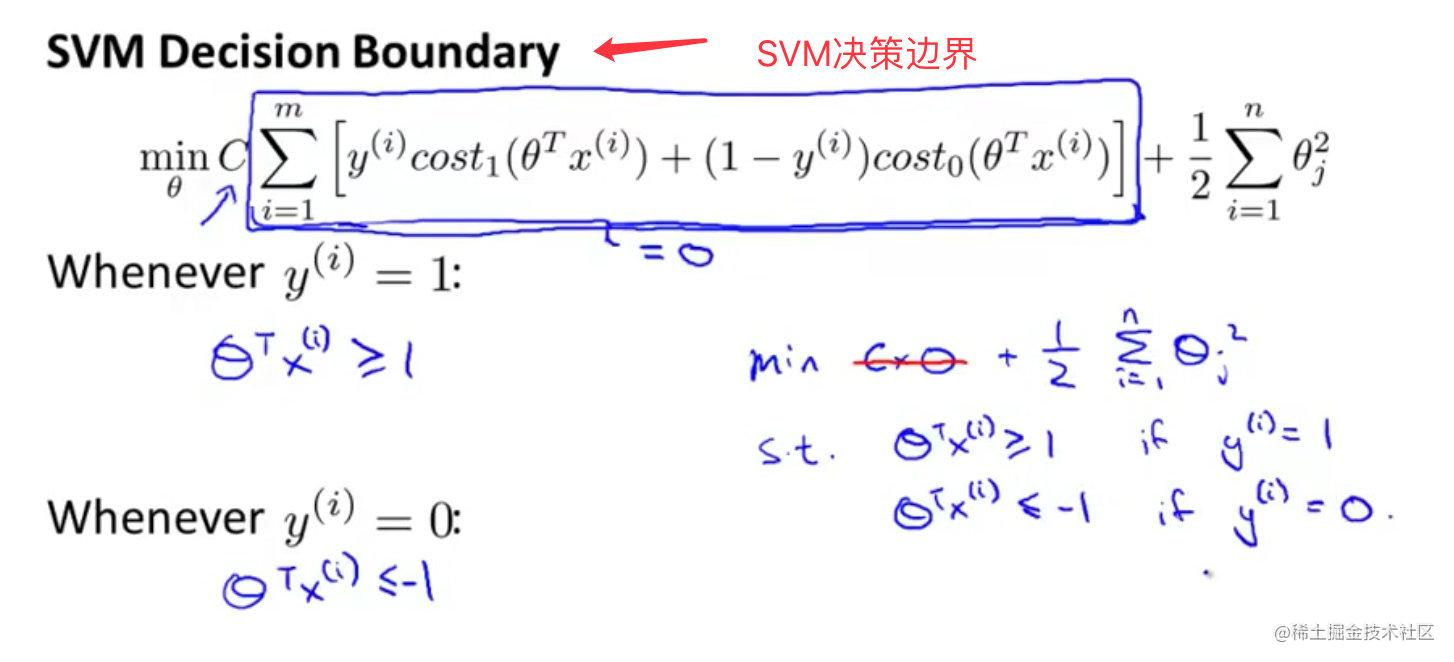SVM鲁棒性：间隔最大化，是一种大间距分类器。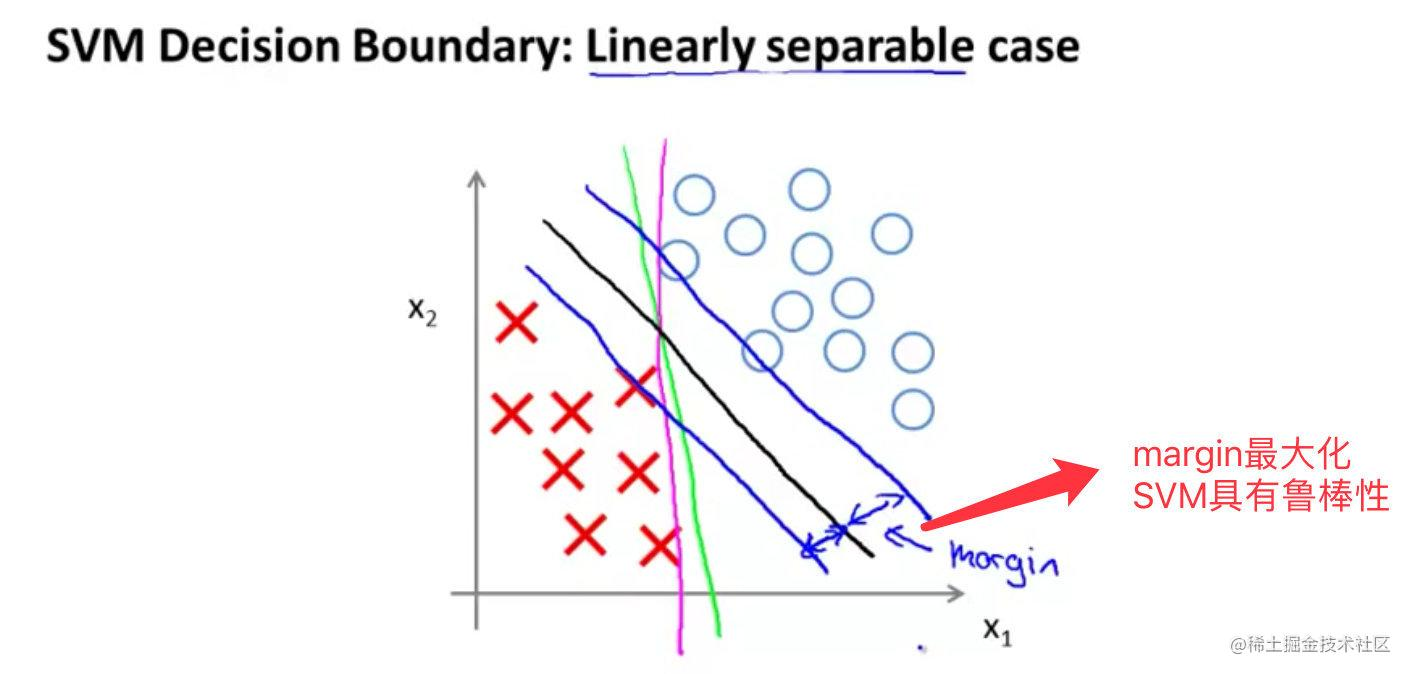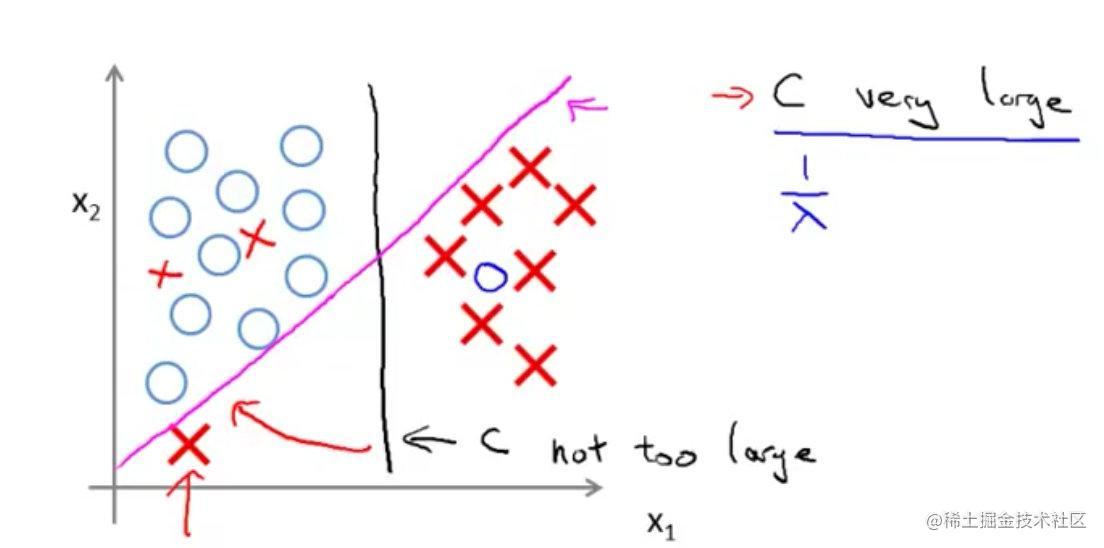1. C太大的话，将是粉色的线
2. C不是过大的话，将是黑色的线

• C较大，可能导致过拟合，高方差
• C较小，可能导致低拟合，高偏差

#### 硬间隔模型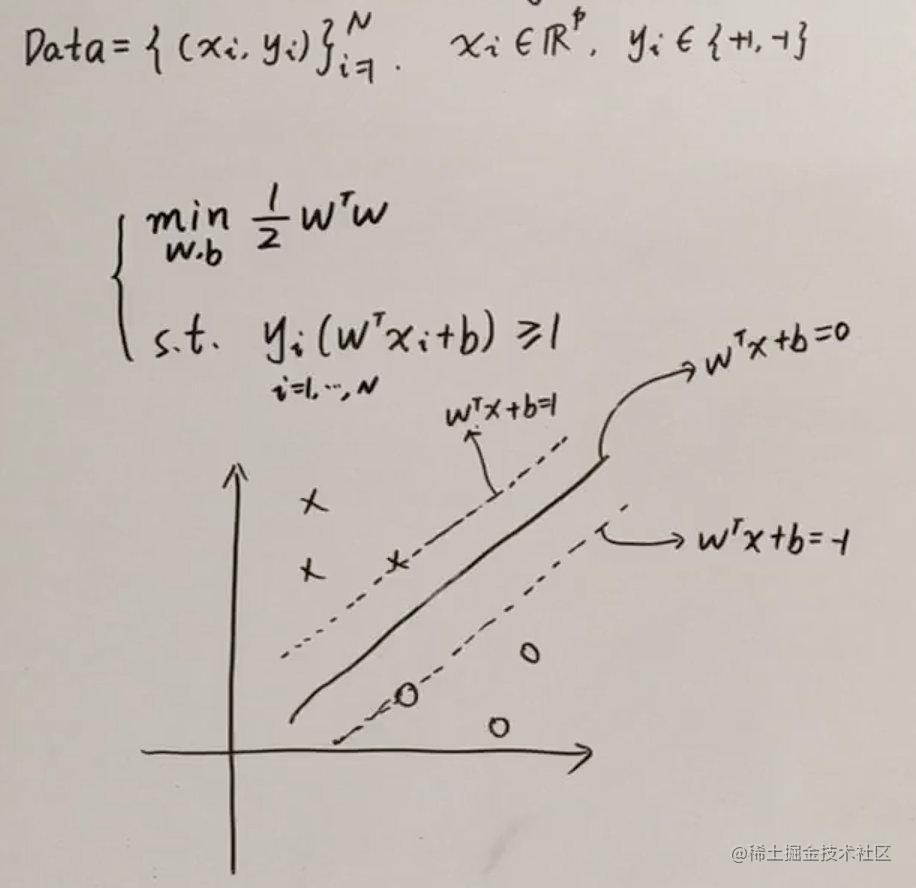### 间隔和支持向量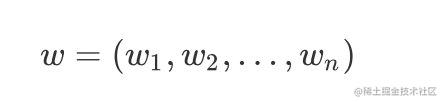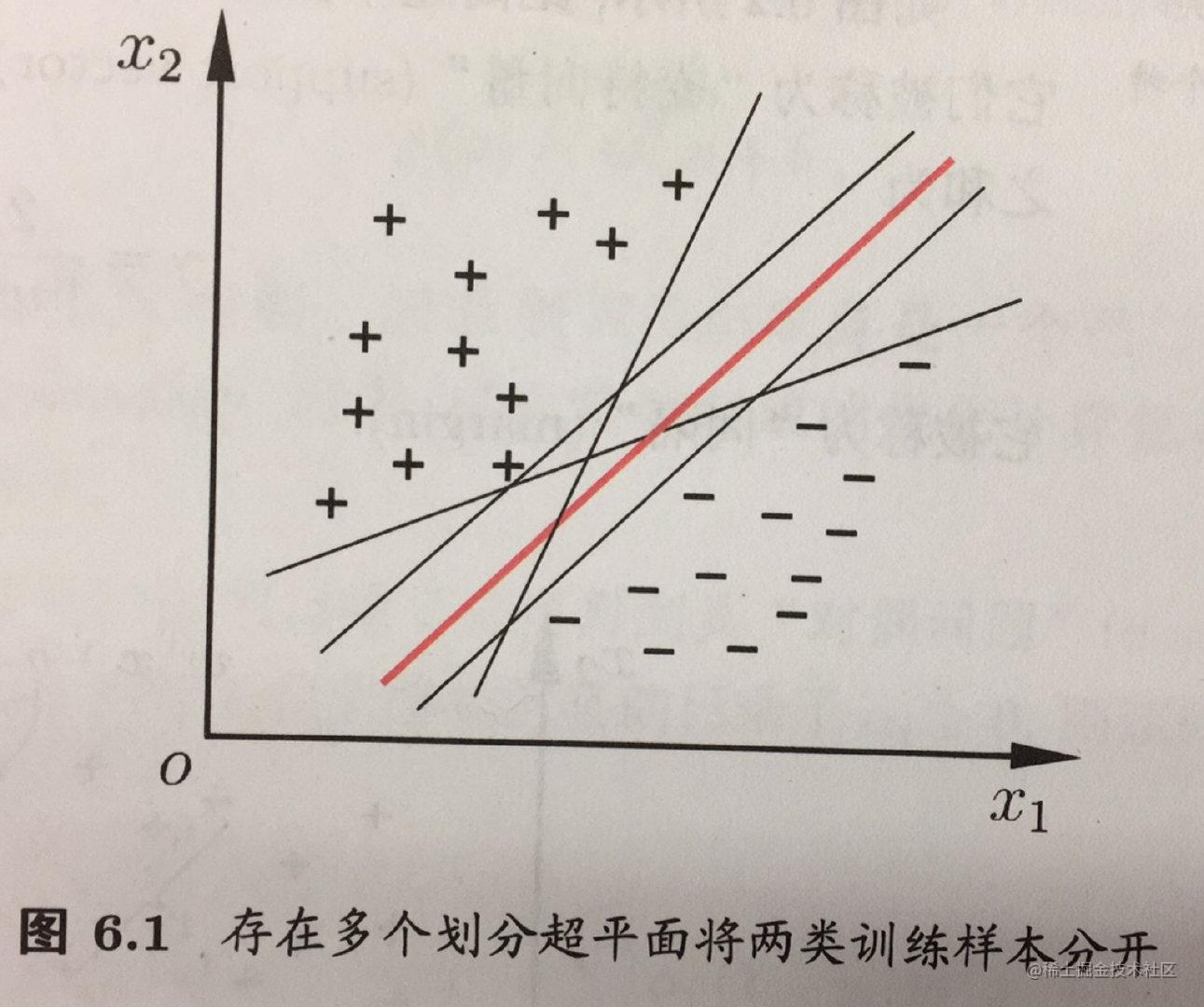W称之为法向量（看做是列向量），决定平面的方向；b是位移项，决定了超平面和原点之间的距离。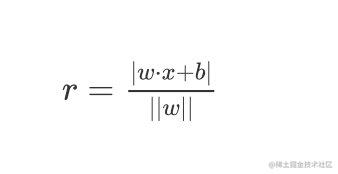$+$区域的点满足$y=+1$$-$区域的点满足$y=-1$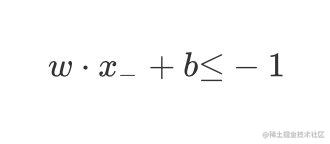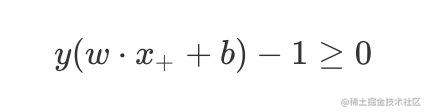#### 支持向量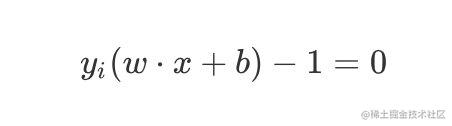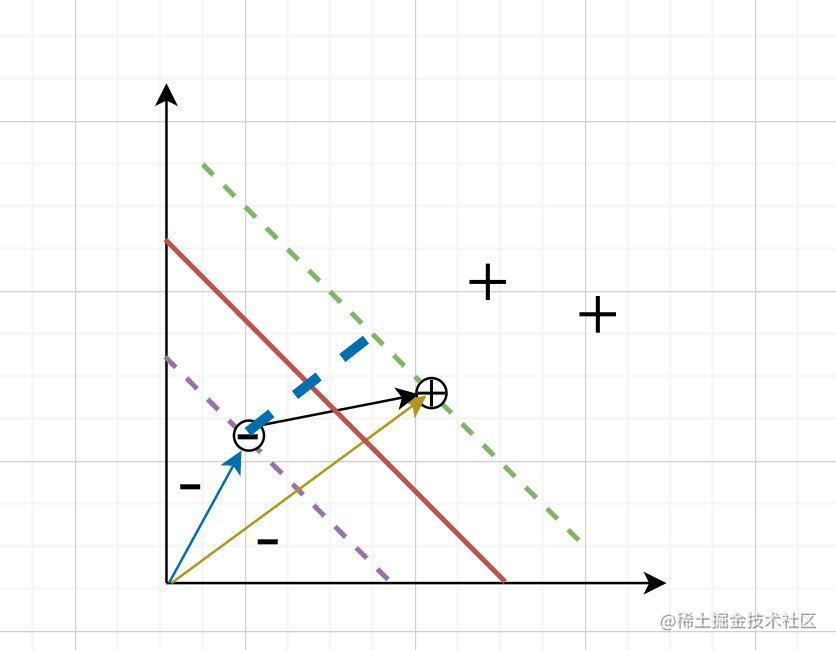#### 间距margin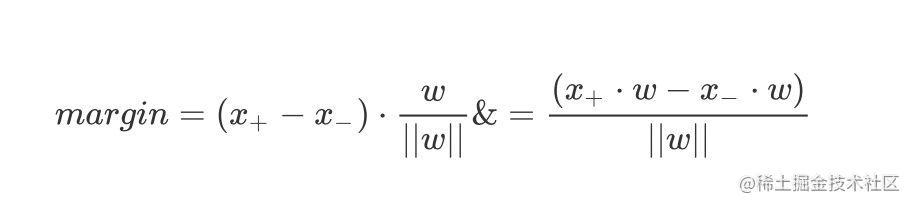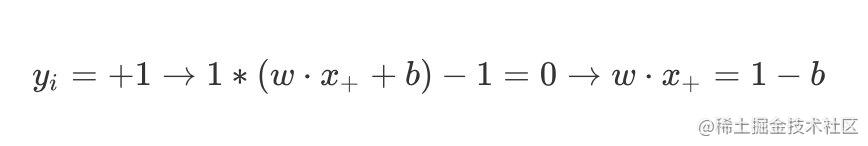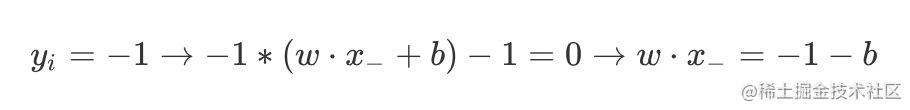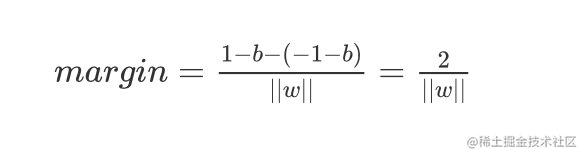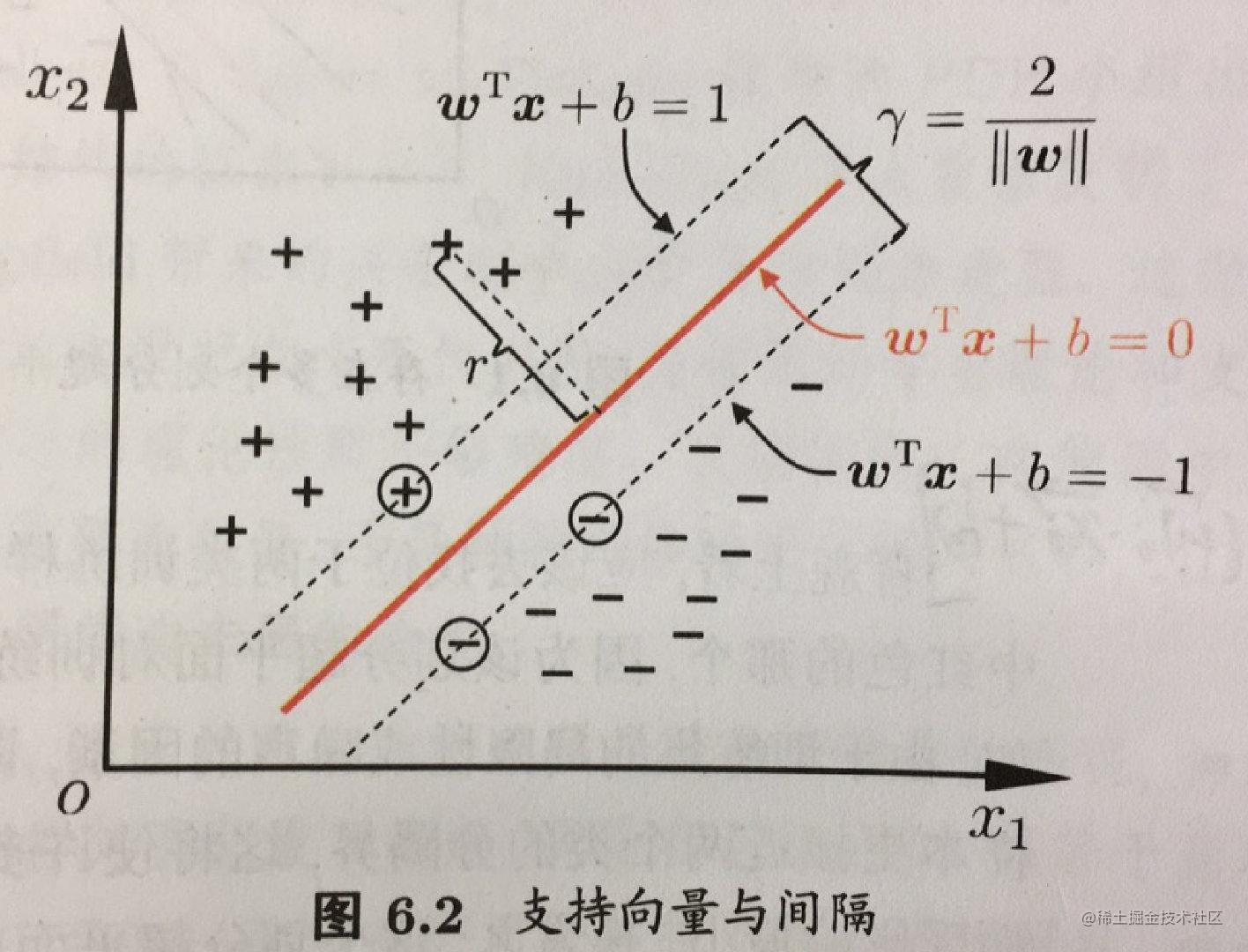#### SVM的基本模型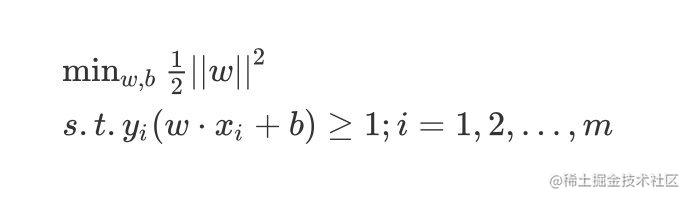### SVM-对偶模型

#### 模型参数推导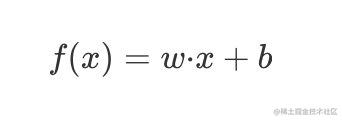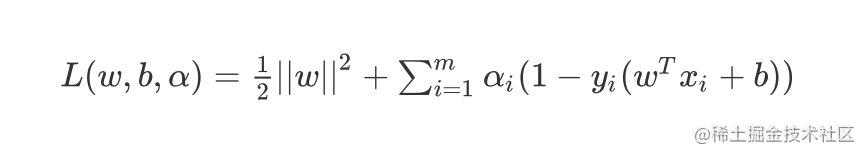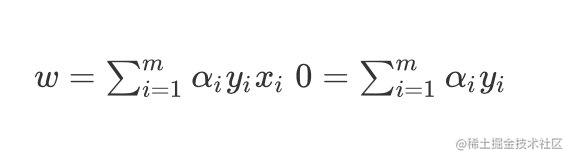#### 对偶模型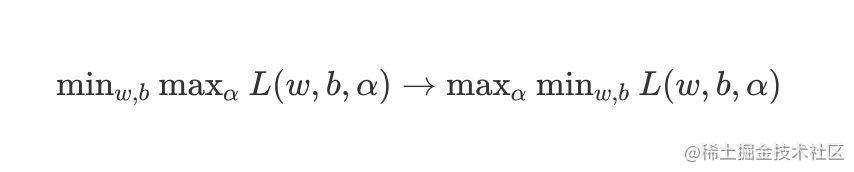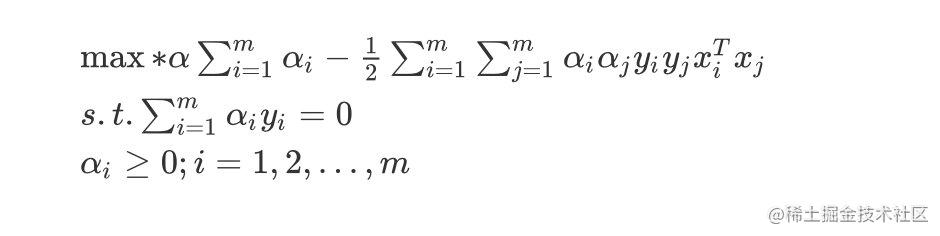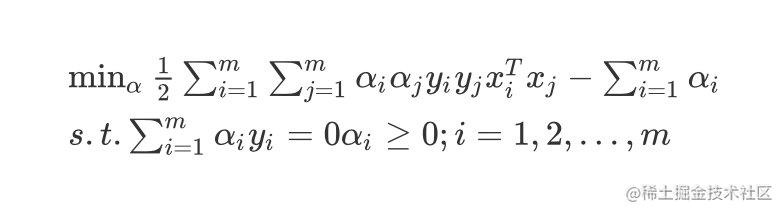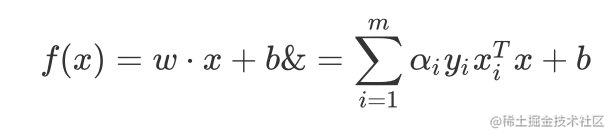### SMO算法

SMO算法指的是Sequential Minimal Optimization，序列最小优化算法。算法的根本思路是：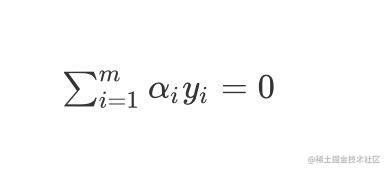1. 先选取需要更新的变量$\alpha_i$$\alpha_j$

2. 固定变量$\alpha_i$$\alpha_j$以外的参数，求解更新后的变量$\alpha_i$$\alpha_j$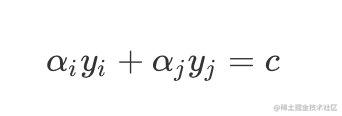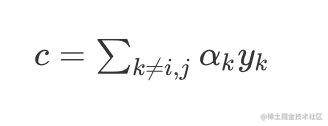1. 将变量$\alpha_i$$\alpha_j$的其中一个用另一个来表示，得到关于$\alpha_i$的单变量二次规划问题，就可以求出来变量$\alpha_i$

### 软间隔最大化